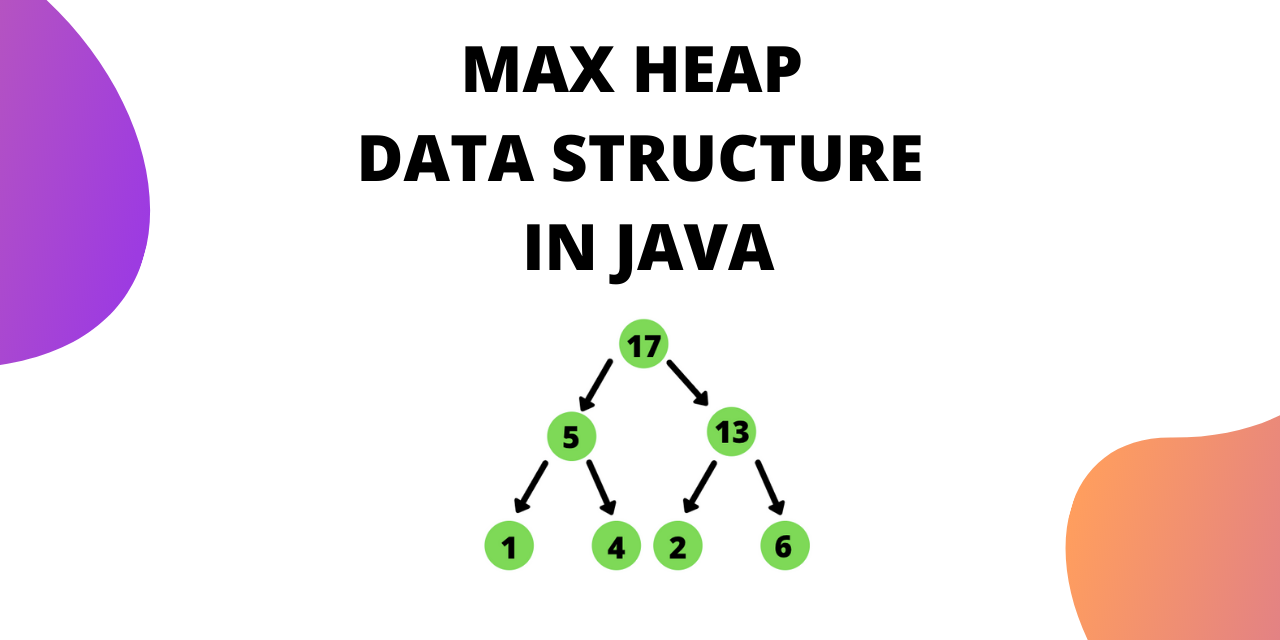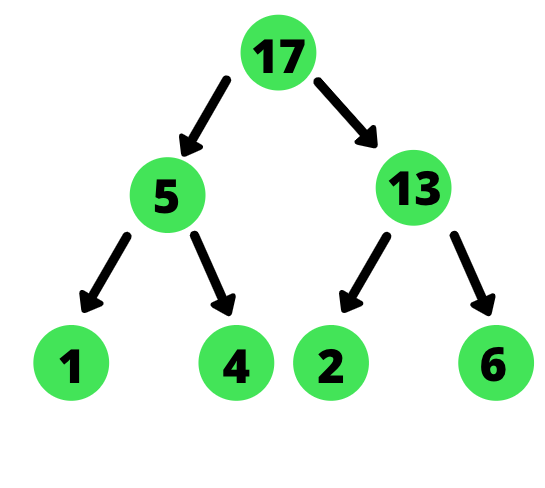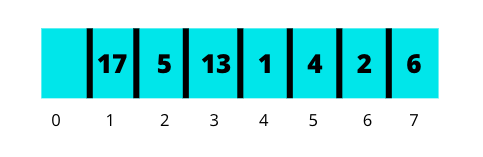# Max Heap Data Structure Implementation in Java

Published on August 3, 2022By Jayant Verma
Developer and author at DigitalOcean.While we believe that this content benefits our community, we have not yet thoroughly reviewed it. If you have any suggestions for improvements, please let us know by clicking the “report an issue“ button at the bottom of the tutorial.

A max heap is a complete binary tree in which the value of a node is greater than or equal to the values of its children. Max Heap data structure is useful for sorting data using heap sort.

In this tutorial, we will cover everything you need to know to implement max heaps in java from scratch.

## Implementation of a Max Heap Data Structure in Java

We represent a heap using an array. Since the heap is a complete binary tree, there is no wastage of space.

For example, let’s consider a heap as follows :The array representation is:The declaration of max heap is done as follows:

`````` static class MaxHeap {
private int[] Heap; // array
private int size;
private int maxsize;

public MaxHeap(int size) {
this.maxsize = size;
this.size = 0;
Heap = new int[this.maxsize + 1];
Heap = Integer.MAX_VALUE;
}
``````

Heap is the array that stores the max heap. The constructor takes the size and initializes the array with 0th element as infinity. We start our heap from index 1.

### 1. Getting parent of a node

Since we are storing the heap as an array, getting the parent for a node becomes easier.

For an element at position i, the position of its parent is given by :

``````(i)/2
``````

During implementation we can get the parent using :

``````private int parent(int pos) {
return pos / 2;
}
``````

### 2. Getting children for the node

For a node at position i, its children are given by the formula :

Left child :

``````(2*i)
``````

Right child :

``````(2*i)+ 1
``````

Note : This is true when your heap is starting from index 1. If the heap is starting at position 0, the values are (2*i) +1 and (2*i) +2 for left and right child respectively.

In code we implement this as follows :

``````  private int leftChild(int pos) {
return (2 * pos) ;
}

private int rightChild(int pos) {
return (2 * pos) + 1;
}
``````

### 3. Heapify a newly inserted element

After inserting an element into the heap, it may not satisfy the heap property. In that case, we need to adjust the locations of the heap to make it heap again. This process is called Heapifying.

To heapify an element in a max heap we need to find the maximum of its children and swap it with the current element. We continue this process until the heap property is satisfied at each node.

In order to heapify we move down from the root to the leaves. Hence this is also known as Down Heapify.

Another interesting point to note is that we perform down heapify only on non-leaf nodes.

The code for down-heapify function is:

`````` private void downHeapify(int pos) {

//checking if the node is a leaf node
if (pos >= (size / 2) && pos <= size)
return;

//checking if a swap is needed
if (Heap[pos] < Heap[leftChild(pos)] ||
Heap[pos] < Heap[rightChild(pos)]) {

//replacing parent with maximum of left and right child
if (Heap[leftChild(pos)] > Heap[rightChild(pos)]) {
swap(pos, leftChild(pos));

//after swaping, heapify is called on the children
downHeapify(leftChild(pos));
} else {

swap(pos, rightChild(pos));
//after swaping, heapify is called on the children
downHeapify(rightChild(pos));
}
}
}
``````

The swap function is as follows:

``````   private void swap(int fpos, int spos) {
int tmp;
tmp = Heap[fpos];
Heap[fpos] = Heap[spos];
Heap[spos] = tmp;
}
``````

You can also write the same code using a while loop instead of recursion.

In down-heapify we were moving from parents towards its children. We can move in a bottom-up fashion as well. When we’re moving in a bottom-up fashion, we compare a node to its parents. This is called Up Heapify.

The code for up-heapify is:

``````  private void heapifyUp(int pos) {
int temp = Heap[pos];
while(pos>0 && temp > Heap[parent(pos)]){
Heap[pos] = Heap[parent(pos)];
pos = parent(pos);
}
Heap[pos] = temp;
}

``````

We’ve written the code for up heapify using a while loop instead of recursion.

### 4. Insert new nodes

New element is added to the end of the array and swaps are performed to make sure that the heap property holds.

The algorithm for insertion is:

1. Increase the heap size
2. Keep the new element at the end of the heap (array)
3. Heapify from bottom to top

The code for insertion is:

`````` public void insert(int element) {
Heap[++size] = element;
int current = size;
heapifyUp(current);
}
``````

### 5. Deleting/extracting nodes

To delete/extract a node from the heap we delete the element from the root. The root always gives the maximum element.

The algorithm for deletion is as follows:

1. Copy the first element into a variable.
2. Copy last element to first position (root).
3. call downHeapify().

The code for deletion is :

``````  public int extractMax() {
int max = Heap;
Heap = Heap[size--];
downHeapify(1);
return max;
}
``````

Here we use size-- to reduce the size of the heap.

## Complete Implementation of Max Heap in Java

The complete java implementation of Max Heap is as follows.

``````package com.JournalDev;

public class Main {
static class MaxHeap {
private int[] Heap;
private int size;
private int maxsize;

public MaxHeap(int size) {
this.maxsize = size;
this.size = 0;
Heap = new int[this.maxsize + 1];
Heap = Integer.MAX_VALUE;
}

private int parent(int pos) {
return pos / 2;
}

private int leftChild(int pos) {
return (2 * pos)  ;
}

private int rightChild(int pos) {
return (2 * pos) + 1;
}

private void swap(int fpos, int spos) {
int tmp;
tmp = Heap[fpos];
Heap[fpos] = Heap[spos];
Heap[spos] = tmp;
}

private void downHeapify(int pos) {
if (pos >= (size / 2) && pos <= size)
return;

if (Heap[pos] < Heap[leftChild(pos)] ||
Heap[pos] < Heap[rightChild(pos)]) {

if (Heap[leftChild(pos)] > Heap[rightChild(pos)]) {
swap(pos, leftChild(pos));
downHeapify(leftChild(pos));
} else {
swap(pos, rightChild(pos));
downHeapify(rightChild(pos));
}
}
}
private void heapifyUp(int pos) {
int temp = Heap[pos];
while(pos>0 && temp > Heap[parent(pos)]){
Heap[pos] = Heap[parent(pos)];
pos = parent(pos);
}
Heap[pos] = temp;
}

public void insert(int element) {
Heap[++size] = element;

int current = size;
heapifyUp(current);

}

public void print() {
for (int i = 1; i <= size / 2; i++) {
System.out.print(+ Heap[i] + ": L- " +
Heap[2 * i] + " R- " + Heap[2 * i + 1]);
System.out.println();
}
}

public int extractMax() {
int max = Heap;
Heap = Heap[size--];
downHeapify(1);
return max;
}
}
public static void main(String[] arg)
{

MaxHeap maxHeap = new MaxHeap(15);
maxHeap.insert(1);
maxHeap.insert(4);
maxHeap.insert(2);
maxHeap.insert(5);
maxHeap.insert(13);
maxHeap.insert(6);
maxHeap.insert(17);

maxHeap.print();
System.out.println("The max is " + maxHeap.extractMax());
}

}

``````
``````Output :

17: L- 5 R- 13
5: L- 1 R- 4
13: L- 2 R- 6
The max is 17
``````

## Conclusion

This tutorial was about Max heap data structure in Java.

Thanks for learning with the DigitalOcean Community. Check out our offerings for compute, storage, networking, and managed databases.Developer and author at DigitalOcean.

#### Still looking for an answer?

Ask a questionSearch for more help

JournalDev
DigitalOcean EmployeeJanuary 15, 2021

This test failed here “assertEquals(4, m.extractMax());” at that point the heap was [MAX, 1,2,4] and position(1) was incorrectly judged to be a leaf if (pos >= (size / 2) && pos = (size i.e. 3/2 =>1) when infact 1 was not a leaf. public void test1(){ MaxHeap m = new MaxHeap(15); m.insert(10); m.insert(9); m.insert(8); m.insert(7); m.insert(6); m.insert(5); m.insert(4); m.insert(13); m.insert(2); m.insert(1); m.insert(17); assertEquals(17, m.extractMax()); assertEquals(13, m.extractMax()); assertEquals(10, m.extractMax()); assertEquals(9, m.extractMax()); assertEquals(8, m.extractMax()); assertEquals(7, m.extractMax()); assertEquals(6, m.extractMax()); assertEquals(5, m.extractMax()); assertEquals(4, m.extractMax()); assertEquals(2, m.extractMax()); assertEquals(1, m.extractMax()); }

- Calvin